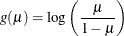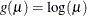Examples of Generalized Linear Models

You construct a generalized linear model by deciding on response and explanatory variables for your data and choosing an appropriate link function and response probability distribution. Some examples of generalized linear models follow. Explanatory variables can be any combination of continuous variables, classification variables, and interactions.

• response variable: a continuous variable

• distribution: normal

• link function: identity,Logistic Regression

• response variable: a proportion

• distribution: binomial

• link function: logit,Poisson Regression in Log-Linear Model

• response variable: a count

• distribution: Poisson

• link function: log,• link function: log,